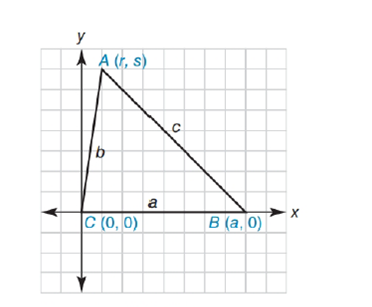Chapter 10.4, Problem 34EElementary Geometry For College St...

7th Edition
Alexander + 2 others
ISBN: 9781337614085

Solutions

Chapter
SectionElementary Geometry For College St...

7th Edition
Alexander + 2 others
ISBN: 9781337614085
Textbook Problem

Complete an analytic proof of the following theorem: In a triangle that has sides of lengths a ,   b , and c , if c 2 = a 2 + b 2 , then the triangle is a right triangle.Exercises 34. 35

To determine

To prove:

A triangle has sides of lengths a, b, and c, if c2=a2+b2, then the triangle is a right triangle

Explanation

Given figure is,

The above figure shows the triangle ABC.

And the coordinates of the quadrilateral ABC is as shown in the figure,

C0, 0, B(a,0) and A(r,s)

a is the length of CB

b is the length of AC

c is the length of AB

a=a-02+(0-0)2

a=a2

c=r-a2+(s-0)2

c=r-a2+s2

b=0-r2+(0-s)2

b=r2+s2

Substituting a, b, c values in the equation c2=a2+b2

Still sussing out bartleby?

Check out a sample textbook solution.

See a sample solution

The Solution to Your Study Problems

Bartleby provides explanations to thousands of textbook problems written by our experts, many with advanced degrees!

Get Started